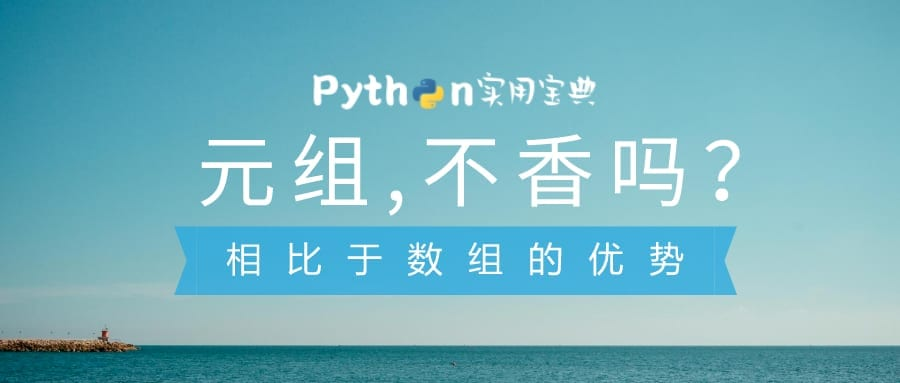# Python 元组Tuple 相对于数组List的优势

## 2.性能快

```>>> import timeit
>>> print(timeit.timeit('["CAR", "AIRPLANE", "BOAT"]'))
0.0508033999999995
>>> print(timeit.timeit('("CAR", "AIRPLANE", "BOAT")'))
0.013855500000001797```

```>>> a_tuple = ("CAR", "AIRPLANE", "BOAT")
>>> b_list = ["CAR", "AIRPLANE", "BOAT"]
>>> from sys import getsizeof
>>> getsizeof(a_tuple)
72
>>> getsizeof(b_list)
88```

## 3.作为函数返回值

```my_tuple = "CAR", "AIRPLANE", "BOAT"
my_tuple2 = ("CAR", "AIRPLANE", "BOAT")

print(type(my_tuple)) # <class 'tuple'>
print(type(my_tuple2)) # <class 'tuple'> ```

​Python实用宝典 (pythondict.com)